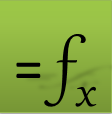# Numerical Integration Functions in Excel

Integrate formulas and functions

Use QUADF to compute a proper or improper integral of any formula or VBA user defined function using highly accurate adaptive algorithms.

Integrate discrete data points sets

Use QUADXY to integrate a discrete set of (x,y) data points using linear or cubic splines.

Compute multiple integrals

Nest QUADF to compute multiple integrals of any order with individualized control of each nested integral such as algorithm selection.

## Description

This video demonstrates how to use the integration functions QUADF for computing accurate integrals in both Excel and Google Sheets. Several examples are solved including fixed limits, infinite limits and singular points. The video also demonstrates how to nest QUADF to compute multiple integrals of any order.

## Description

This video demonstrates how to use the function QUADXY to compute accurate integral of a graph generated from discrete (x,y) data set points in both Excel and Google Sheets by the aid of splines.
Question or Comment? Email us:
support @ excel-works.comExceLab: Transforming Excel into a Calculus Power House

ExceLab functions and methods are protected by USA Patents 10628634, 10114812, 9892108 and 9286286.